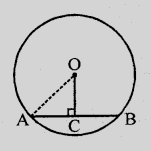# The radius of a circle is $8\ cm$ and the length of one of its chords is $12\ cm$. Find the distance of the chord from the centre.

Given:

The radius of a circle is $8\ cm$ and the length of one of its chords is $12\ cm$.

To do:

We have to find the distance of the chord from the centre.

Solution:

Let the radius of the circle with centre $O$ be $OA = 8\ cm$

Length of the chord $AB = 12\ cm$$OC \perp AB$ which bisects $AB$ at $C$

Therefore,

$AC = CB = 12 \times \frac{1}{2} = 6\ cm$

In $\triangle OAC$,

$OA^2 = OC^2 + AC^2$               (Pythagoras Theorem)

$(8)^2 = OC^2 + (6)^2$

$64 = OC^2 + 36$

$OC^2 = 64 - 36 = 28$

$\Rightarrow OC = \sqrt{28}$

$= 2\sqrt{7}\ cm$

$= 2 \times 2.6457$

$= 5.291\ cm$

The distance of the chord from the centre is $5.291\ cm$.

Updated on: 10-Oct-2022

208 Views